# Excercise 5.1 Lines-and-Angles - NCERT Solutions Class 7

## Chapter 5 Ex.5.1 Question 1

Find the complement of each of the following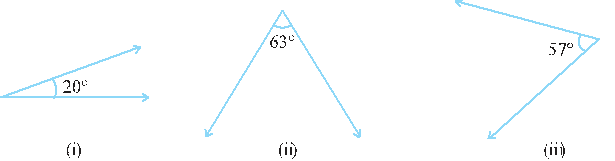### Solution

What is known?

Measure of angles.

What is the unknown?

Measure of complementary angles.

Reasoning:

The sum of complementary angles is always If the given angle is $$x$$, then we can find complementary angle by subtracting from . Let us now find complement angles.

Steps:

i) Given angle $$=20^\circ$$

Complement angle of $$20^\circ$$

\begin{align} &= 90^{\circ} -\text{[given angle]} \\ &=90^{\circ}- 20^{\circ} \\ &=70^{\circ} \end{align}

(ii) Given angle  $$= 63^\circ$$

Complement angle of $$63^\circ$$

\begin{align} &= 90^{\circ} -\text{[given angle]} \\ &= 90^{\circ}-63^{\circ} \\ &= 27^{\circ} \end{align}

(iii) Given angle  $$= 57^\circ$$

Complement angle of $$57^\circ$$

\begin{align} &= 90^{\circ}- \text{[given angle]} \\ &= 90^{\circ}-57^{\circ} \\ &= 33^{\circ} \end{align}

## Chapter 5 Ex.5.1 Question 2

Find the supplement of each of the following angles: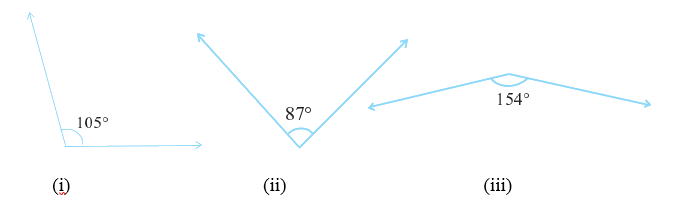### Solution

What is known?

Measure of angles.

What is the unknown?
Measure of supplement of each angle.

Reasoning:
In a pair of angles, if the sum of the measures of the angles comes out to be $$180^\circ$$, angles are called supplementary angles. When two angles are supplementary, each angle is said to be the supplement of the other. If the given angle is $$x$$, then we can find the supplement by subtracting $$x$$ from $$180^\circ$$.

Steps:
(i) Given angle $$=105^\circ$$

Supplement angle of $$105^\circ$$

\begin{align} &= {180^\circ} - \text{given angle} \\ &= 180^\circ − 105^\circ \\ &= 75^\circ \end{align}

(ii) Given angle $$= 87^\circ$$

Supplement angle of $$87^\circ$$

\begin{align} &= 180^\circ -\text{given angle} \\ &= 180^\circ − 87^\circ \\ &= 93^\circ \end{align}

(iii) Given angle $$=154^\circ$$

Supplement angle of $$154^\circ$$

\begin{align} &= 180^\circ -\text{given angle} \\ &= 180^\circ − 154^\circ \\ &=26^\circ\end{align}

## Chapter 5 Ex.5.1 Question 3

Identify which of the following pairs of angles are complementary and which are supplementary:

(i) $$65^\circ, 115^\circ$$

(ii) $$63^\circ, 27^\circ$$

(iii) $$112^\circ, 68 ^\circ$$

(iv) $$130^\circ, 50^\circ$$

(v) $$45^\circ, 45^\circ$$

(vi) $$80^\circ, 10^\circ$$

NOTE: The sum of the measure of complementary angle is $$90^\circ$$ and that of supplementary angle is $$180^\circ$$

### Solution

Reasoning:

Find out the sum of two given angles,and then check whether it is $$180^\circ$$ or $$90^\circ.$$ If  the sum of two angles is either equal to $$90^\circ$$, the angles are complementary and if the sum of the two angles is $$180^\circ$$, the angles are complementary.

Steps:

Solve for supplementary angle or complementary angle:

(i) $$65^\circ, 115^\circ$$

Sum of measure of these two angles $$= 65^\circ + 115^\circ = 180^\circ$$

Therefore, these two angles are supplementary.

(ii) $$63^\circ, 27^\circ$$

Sum of measure of these two angles $$= 63^\circ + 27^\circ = 90^\circ$$

Therefore, these two angles are complementary.

(iii) $$112^\circ, 68^\circ$$

Sum of measure of these two angles $$= 112^\circ + 68^\circ = 180^\circ$$

Therefore, these two angles are supplementary.

(iv) $$130^\circ, 50^\circ$$

Sum of measure of these two angles $$= 130^\circ + 50^\circ = 180^\circ$$

Therefore, these two angles are supplementary.

(v) $$45^\circ, 45^\circ$$

Sum of measure of these two angles $$= 45^\circ + 45^\circ = 90^\circ$$

Therefore, these two angles are complementary.

(vi) $$80^\circ, 10^\circ$$

Sum of measure of these two angles $$= 80^\circ + 10^\circ = 90^\circ$$

Therefore, these two angles are complementary.

## Chapter 5 Ex.5.1 Question 4

Find the angle which is equal to its complement.

### Solution

What is known?

An angle which is equal to its complement.

What is the unknown?

Find measure of the angle.

Reasoning:

Let us say given angle is $$x$$ and according to the question, its complement angle will also be equal to $$x$$. The sum of angle and its complement angle is always equal to $$90^\circ.$$

Steps:

Solve for angle which is equal to its complement.

Let the angle be $$x$$.

Therefore, complement of this angle will also be $$x$$.

We know that, the sum of measure of pair of complementary angles is $$90^\circ.$$

\begin{align} x + x &= 90^\circ\\\Rightarrow2x &=90^\circ\\ \,\Rightarrow x &=\frac{90^\circ}2\\\Rightarrow x &=45^\circ\end{align}

Thus, the angle which is equal to its complement is $$45^\circ.$$

## Chapter 5 Ex.5.1 Question 5

Find the angle which is equal to its supplement.

### Solution

What is known?

An angle which is equal to its supplement.

What is the unknown?

Find measure of the angle.

Reasoning:

Let us say given angle is $$x$$  and according to the question, its supplementary angle will also be equal to $$x$$. The sum of angle and its supplement angle is always equal to $$180^\circ.$$

Steps:

Let the angle be $$x.$$

Therefore, supplement of this angle will also be $$x.$$

We know that, the sum of measure of pair of supplementary angles is $$180 \,^{\circ}$$

\begin{align} x+x &=180^\circ\\ \Rightarrow2x &=180^\circ\\ \Rightarrow x&=(180^\circ)/2\\ \Rightarrow x&=90^\circ\end{align}

Thus, the angle which is equal to its supplement is $$90^\circ.$$

## Chapter 5 Ex.5.1 Question 6

In the given figure, $$\angle 1$$ and $$\angle 2$$ are supplementary angles. If  $$\angle 1$$ is decreased, what changes should take place in $$\angle 2$$ so that both the angles remain supplementary?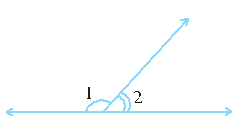### Solution

What is known?

Two angles which are equal to its supplementary.

What is the unknown?

Change in one of the angles if other is decreased provided both angles still remain supplementary.

Reasoning:

Let’s solve this problem visually. There are two operations done in sequence. First, if one angle is decreasing then another angle will increase but their sum will remain same i.e. $$180^\circ.$$

According to this model, the resultant sum of angle $$\angle 1$$ and $$\angle 2$$ will remain $$180^\circ.$$

Steps:

If $$\angle 1$$ is decreased by some degrees, then $$\angle 2$$ will also be increased with the same degree, so both the angles will remain supplementary.

## Chapter 5 Ex.5.1 Question 7

Can two angles be supplementary if both are:

(i) acute

(ii) obtuse

(iii) right

### Solution

Steps:

(i) No, sum of acute angles is less than  $$180^\circ.$$

(ii) No, sum of obtuse angles is greater than $$180^\circ.$$

(iii) Yes, sum of two right angles is $$180^\circ.$$

## Chapter 5 Ex.5.1 Question 8

An angle is greater than $$45^\circ.$$ Is its complementary angle greater than $$45^\circ$$ or equal to $$45^\circ$$ or less than $$45^\circ\,?$$

### Solution

Reasoning:

Let us represent the angle by $$\angle 1$$ and its complement angle by$$\angle 2.$$ Let’s visually model this problem. There are two operations done in sequence. First take$$\angle 1\,\, \gt 45^\circ$$ as it is given, and then add $$\angle 2$$ to both sides of the equation.

According to this model, the result is equal to $$\angle 1 + \angle 2\,\, \gt 45 ^\circ + \angle 2.$$ Now, it’s a matter of finding Is its complementary angle greater than $$45^\circ$$ or equal to $$45^\circ$$ or less than $$45^\circ.$$

Steps:

Let there be two angles $$\angle 1$$ and $$\angle 2 .$$

Therefore$$\angle 1 \gt 45^\circ$$ (given)

Adding $$\angle 2$$  to both sides ,we get

$$=\gt \angle 1 + \angle 2 \gt 45^\circ + \angle 2\\ =\gt 90^\circ \gt 45^\circ + \angle 2 \\ =\gt 90^\circ - 45^\circ \gt \angle 2 \\ =\gt 45^\circ \gt \angle 2$$

Therefore, its complementary angle will be less than $$45^\circ.$$

## Chapter 5 Ex.5.1 Question 9

In the adjoining figure:

(i) Is $$\angle 1$$ adjacent to $$\angle 2$$ $$?$$

(ii) Is $$\angle AOC$$ adjacent to $$\angle AOE \,?$$

(iii) Do $$\angle COE$$ and $$\angle EOD$$ form a linear pair?

(iv) Are $$\angle BOD$$ and $$\angle DOA$$supplementary?

(v) Is $$\angle 1$$ vertically opposite to $$\angle 4\,?$$

(vi) What is the vertically opposite angle of $$\angle 5\,?$$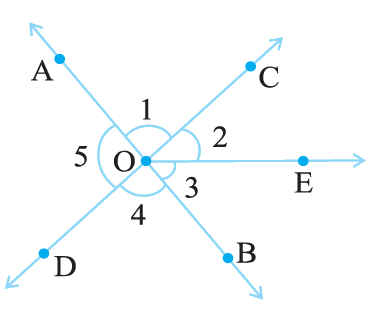### Solution

Steps:

(i) Yes, because they have common vertex $$O$$ and common arm $$OC.$$

(ii) No, they have non-common arms on either  side of common arms.

(iii) Yes, they form linear pair.

(iv) Yes, they are supplementary.

(v) Yes, they are vertical angles because they are formed due to intersection of straight lines.

(vi) Vertically opposite angle of $$\angle 5$$ is $$\angle 2 + \angle 3$$ i.e, $$\angle COB.$$

## Chapter 5 Ex.5.1 Question 10

Indicate which pairs of angles are:

(i) Vertically opposite angles?

(ii) Linear pairs?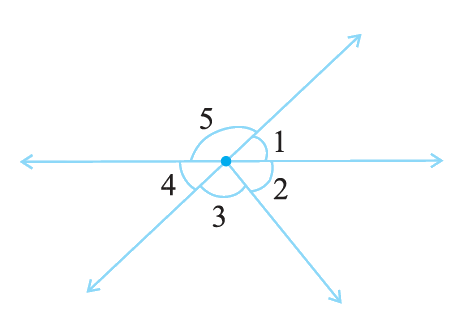### Solution

Steps:

(i) $$\angle 1$$ and $$\angle 4, \angle 5$$ and ( $$\angle 2+\angle 3$$) are vertically opposite angles as they formed due to intersection of two straight lines.

(ii) $$\angle 1$$ and$$\angle 5 , \angle 5$$ and $$\angle 4$$ forms linear pair.

## Chapter 5 Ex.5.1 Question 11

In the following figure, is $$\angle 1$$ adjacent to $$\angle 2?$$ Give reasons.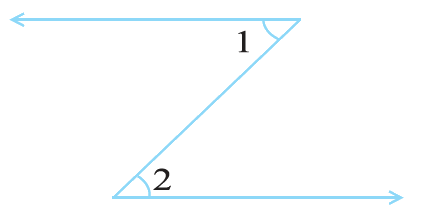### Solution

Steps:

$$\angle 1$$ is not adjacent to $$\angle 2$$ because their vertex is not common.

## Chapter 5 Ex.5.1 Question 12

Find the values of the angles $$x, y$$ and $$z$$ in each of the following: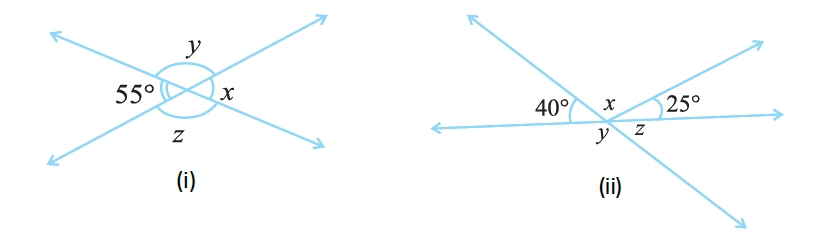### Solution

(i) Reasoning

There are two operations done in sequence. First, if one angle is $$55^\circ$$ then the angle opposite to it will also be $$55^\circ$$as vertically opposite angles are equal.

Also sum of $$\angle x + \angle y = 180^\circ$$ and $$\angle z + 55^\circ = 180^\circ.$$ Now, $$\angle x,\angle y$$ and $$\angle z$$ can be easily calculated.

Steps:

Solve for $$\angle x,\angle y$$ and $$\angle z:$$

(i) $$\angle x = 55^\circ$$ (Vertically opposite angle)

\begin{align} \angle x + \angle y &= 180^\circ \rm (Linear \,pair) \\55 ^\circ+ \angle y &= 180^\circ\\ \angle y &= 180^\circ- 55 ^\circ\\ \angle y &= 125 ^\circ\end{align}

Therefore$$\angle y= \angle z = 125^\circ$$ (Vertically opposite angle)

Hence, $$\angle x = 55^\circ,\angle y= 125^\circ,\angle z = 125^\circ$$

(ii) Reasoning

By using angle sum property find the value of $$x$$ and then find the value of $$y$$ and $$z.$$ Since the sum of $$y + z = 180^\circ.$$ Now, it’s a matter of finding $$y$$ and $$z.$$

By using angle sum property,

\begin{align}40^\circ \!+ \!x \!+ \!25^\circ &= 180^\circ \\ \text{(Angles on } &\text{straight line)}\\x + 65^\circ &= 180^\circ\\ x &= 180^\circ - 65^\circ = 115^\circ\end{align}

Also,

\begin{align}40^\circ + y &= 180^\circ\text{(Linear pair)}\\ y &= 180^\circ - 40^\circ\\ y &= 140^\circ\\y + z &= 180^\circ \text{(Linear pair)}\\140^\circ + z &= 180^\circ (y = 140^\circ)\\ z &= 180^\circ- 140^\circ\\ z &= 40^\circ \end{align}

Thus, $$x = 115^\circ,y = 140^\circ {\text {and}} \,\,z = 40^\circ$$

## Chapter 5 Ex.5.1 Question 13

Fill in the blanks:

(i) If two angles are complementary, then the sum of their measures is _____________.

(ii) If two angles are supplementary, then the sum of their measures is _____________.

(iii) Two angles forming a linear pair are _______________.

(iv) If two adjacent angles are supplementary, they form a _______________.

(v) If two lines intersect a point, then the vertically opposite angles are always

(vi) If two lines intersect at a point and if one pair of vertically opposite angles are acute angles, then the other pair of vertically opposite angles are ______________

### Solution

Steps:

(i)   $$90^\circ$$

(ii)  $$180^\circ$$

(iii) Supplementary

(iv) Linear Pair

(v)  Equal

(vi) Obtuse angle

## Chapter 5 Ex.5.1 Question 14

In the adjoining figure, name the following pairs of angles:

(i) Obtuse vertically opposite angles.

(ii) Adjacent complementary angles

(iii) Equal supplementary angles.

(iv) Unequal supplementary angles.

(v) Adjacent angles that do not form a linear pair.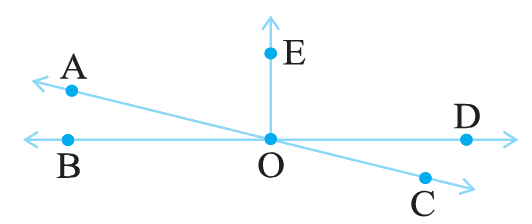### Solution

Steps:

(i) Obtuse vertically opposite angles mean angles greater than $$90^\circ$$ and are equal

$$\angle AOD = \angle BOC$$

(ii) Adjacent complementary angles have common vertex and common arm, non - common arms are on either sides of common arm and their sum is $$90^\circ.$$

$$\angle EOA \,\,{\text {and}} \,\,\angle AOB$$ are adjacent complementary angles.

(iii) Equal supplementary angles have sum of angles $$180^\circ$$ and supplementary angles are equal.

$$\angle EOB\,\,{\text {and} }\,\,\angle EOD$$

(iv) Unequal supplementary angles have sum of angles $$180^\circ$$ and supplementary angles are unequal.

$$\angle EOA$$ and  $$\angle EOC.$$

(v) Adjacent angles that do not form a linear pair. (Linear pairs are adjacent angles whose sum is equal to $$180^\circ$$).

$$\angle AOB$$ and $$\angle AOE;$$ $$\angle AOE$$ and $$\angle EOD;$$ $$\angle EOD$$ and $$\angle COD$$

Instant doubt clearing with Cuemath Advanced Math Program